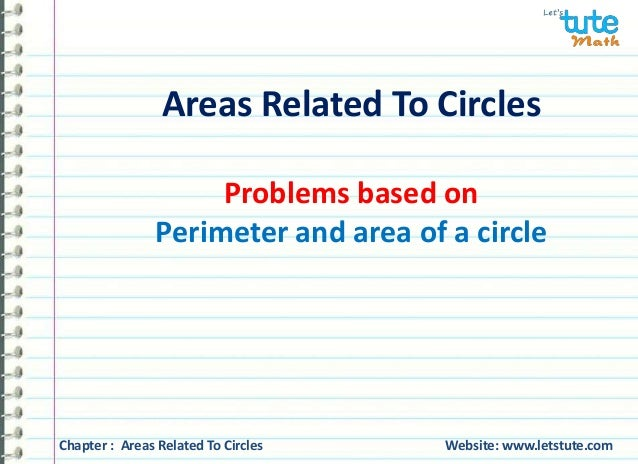Successfully reported this slideshow.Upcoming SlideShare
×

# Areas related to circles - perimeter and area of a circle for class 10 maths.

2,024 views

Published on

In this video you will learn Areas related to circles - perimeter and area of a circle

To watch more such videos -

Visit - www.letstute.com

Thank you

Published in: Education
• Full Name
Comment goes here.

Are you sure you want to Yes No### Areas related to circles - perimeter and area of a circle for class 10 maths.

1. 1. Areas Related To Circles Problems based on Perimeter and area of a circle Chapter : Areas Related To Circles Website: www.letstute.com
2. 2. Problems based on Perimeter and area of a circle Q) Find the circumference and area of the circle of radius 7.2cm. Given: Radius of the circle = 7.2cm To Find: a) Circumference of the circle = ? b) Area of the circle = ? O 7.2 cm Chapter : Areas Related To Circles Website: www.letstute.com
3. 3. Problems based on Perimeter and area of a circle Solution: Let the radius of the circle be ‘r’ r=7.2cm ---------- (given) a) Circumference = 2πr =2x22x7.2cm 7 = 45.26 cm b) Area = πr2 = 22x7.2x7.2cm2 7 = 162.93 cm2 O 7.2 cm Chapter : Areas Related To Circles Website: www.letstute.com
4. 4. Problems based on Perimeter and area of a circle Result: a) Circumference= 45.26cm b) Area= 162.93cm2 Hence, the circumference of the circle is and its area is 45.26cm 162.93 cm2 O 7.2 cm Chapter : Areas Related To Circles Website: www.letstute.com
5. 5. Problems based on Perimeter and area of a circle Q) The diameter of a watermelon slice is 8cm. Find its perimeter. Given: Diameter = 8cm To Find: Perimeter of the slice = ? 8cm Chapter : Areas Related To Circles Website: www.letstute.com
6. 6. Problems based on Perimeter and area of a circle Solution: Let ‘r’ be the radius of the watermelon slice. 8cm r = Diameter = 8cm = 4cm 2 2 Perimeter = 1x 2πr+2r of the slice 2 22 1     4  2 4   2 2 =20.57 cm    7 20.57cm Result: Perimeter of the slice = 20.57 cm Hence, the perimeter of the watermelon slice is Chapter : Areas Related To Circles Website: www.letstute.com
7. 7. Problems based on Perimeter and area of a circle Q) The difference between the circumference and diameter of a wheel is 18cm. Find the radius of the wheel. Given: Circumference - diameter=18cm To Find: Radius of the wheel = ? O ? cm Chapter : Areas Related To Circles Website: www.letstute.com
8. 8. Problems based on Perimeter and area of a circle Solution: Let the radius of the wheel be ‘r’ Circumference-Diameter = 18cm ------- (Given) 2πr-2r = 18cm 2r(π-1) = 18cm 2r  1  = 18cm  2r  = 18cm 2r x 15 = 18cm     22 7 7 7 r = 18x7 cm 2x15    22 7   O ? cm Chapter : Areas Related To Circles Website: www.letstute.com
9. 9. Perimeter and area of a circle = 9x7 cm 15 = 3x7 cm 5 = 21 cm 5 = 4.2cm Problems based on Result: Radius of the wheel= 4.2cm Hence, the radius of the wheel is 4.2cm O ? cm Chapter : Areas Related To Circles Website: www.letstute.com
10. 10. Problems based on Perimeter and area of a circle Q) A wire is in the form of a circle of radius 50cm. It is bent into a square. Determine the side of the square. O Before Given : Radius of the circle = 50 cm To Find: Side of the square = ? Chapter : Areas Related To Circles Website: www.letstute.com
11. 11. Problems based on Perimeter and area of a circle Solution: Let the radius of the circle be ‘r’ r = 50cm ---------- (given) Length of the wire = Circumference of the circle = 2πr = 2x22x50cm 7 = 2200 cm 7 = 314.28cm O Before Chapter : Areas Related To Circles Website: www.letstute.com
12. 12. Problems based on Perimeter and area of a circle Let ‘x’ be the side of the square. Perimeter of the square = Length of the wire (circumference) 4x = 314.28 x = 314.28 4 x = 78.57 cm X cm After Hence, the side of the square is 78.57cm Chapter : Areas Related To Circles Website: www.letstute.com
13. 13. Visit us on – www.letstute.com Subscribe us on www.youtube.com/letstute Thank you